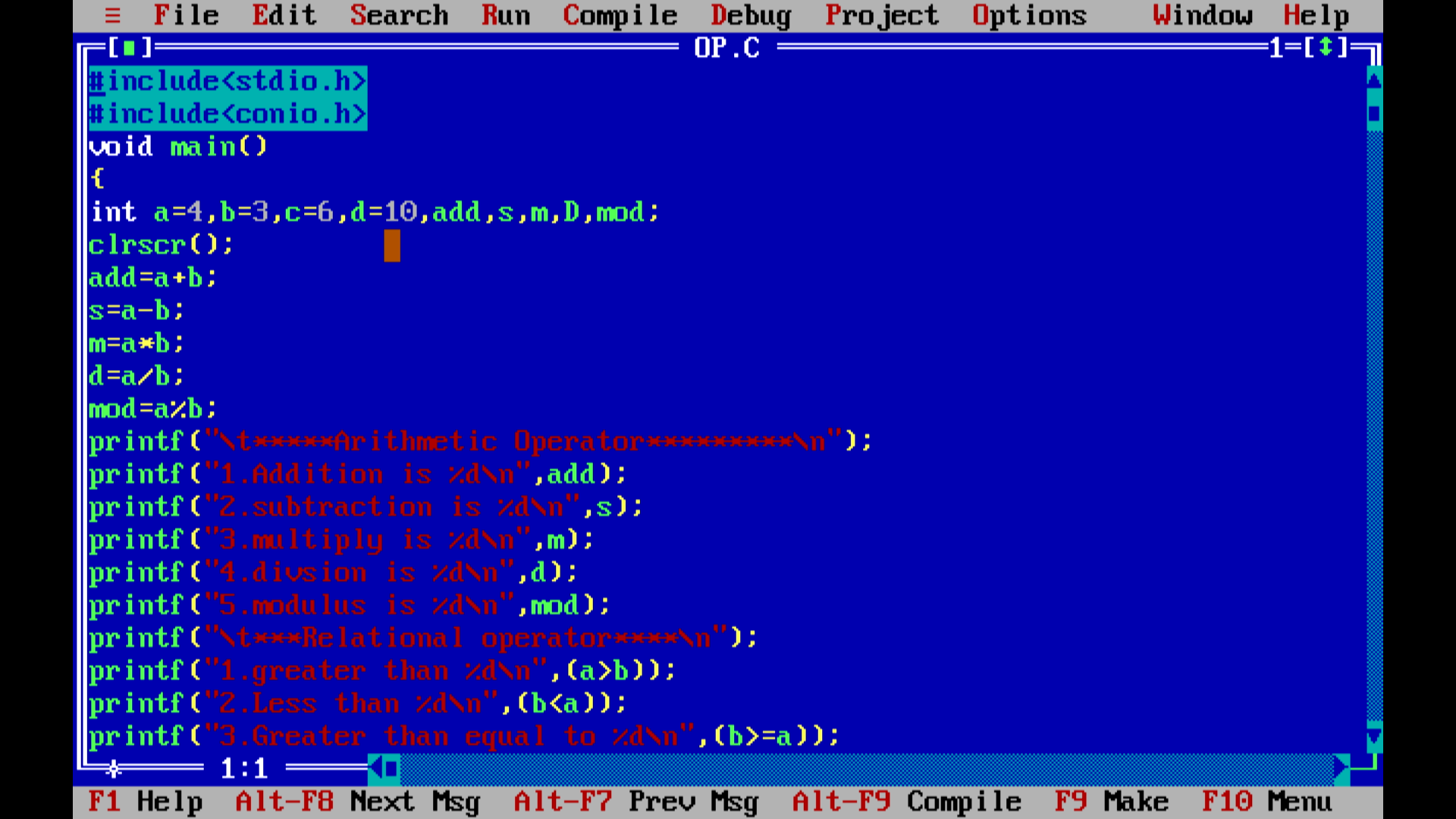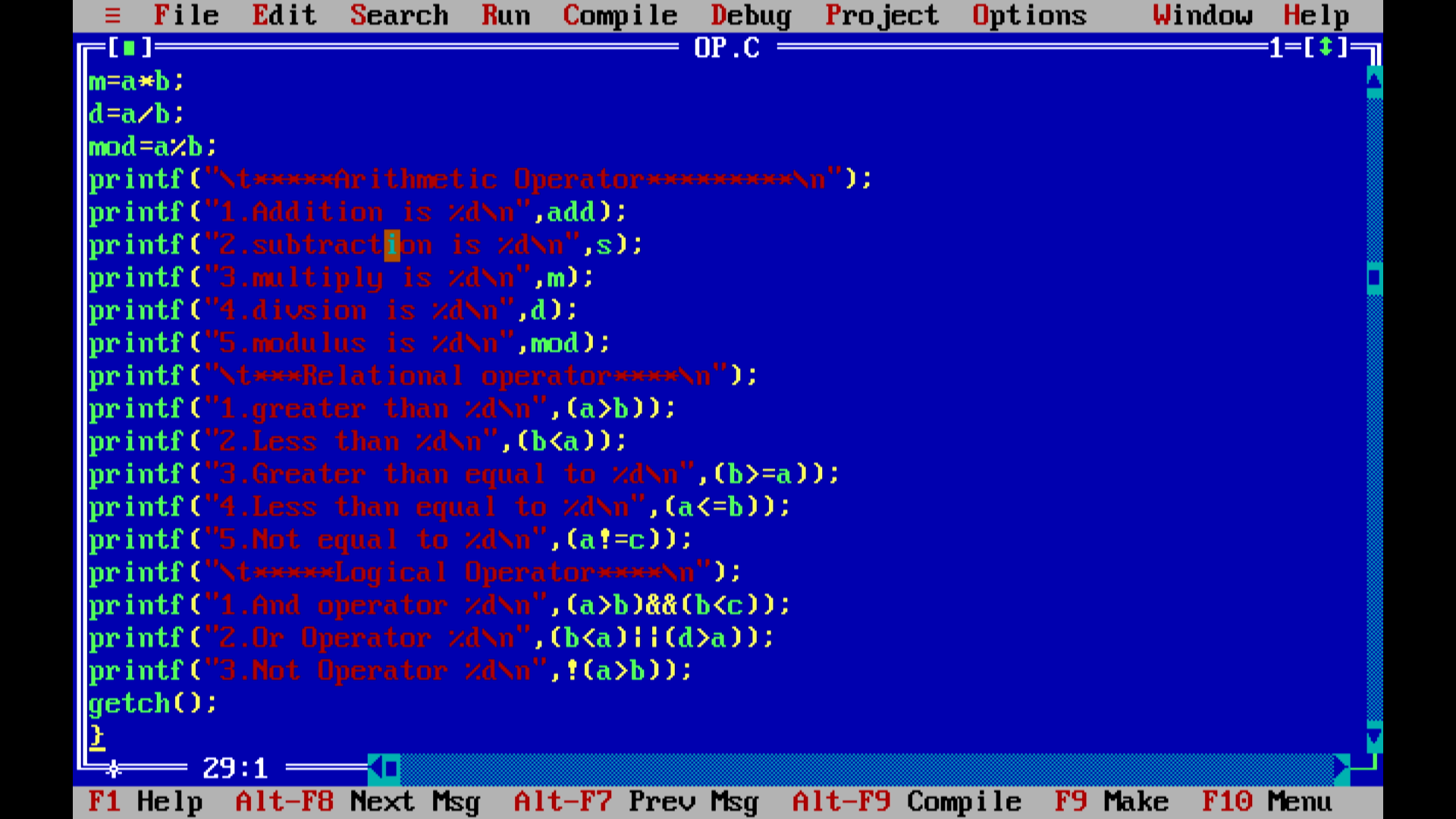Article

# Chapter-4 (Operators in C)

In the C programming language, operators are special symbols or keywords that perform operations on operands. Operands are the values or variables that operators act upon. C provides a wide range of operators that can be categorized into the following groups:

1.Arithmetic Operators:
• Subtraction -
• Multiplication *
• Division /
• Modulus % (remainder)
2.Relational Operators:
• Equal to ==
• Not equal to !=
• Greater than >
• Less than <
• Greater than or equal to >=
• Less than or equal to '<='
3.Logical Operators:
• Logical AND &&
• Logical OR ||
• Logical NOT !
4.Assignment Operators:
• Assignment =
• Subtract and assign -=
• Multiply and assign *=
• Divide and assign /=
• Modulus and assign %=
5. Increment and Decrement Operators:
• Increment ++
• Decrement --
6. Conditional (Ternary) Operator:
• Conditional expression ? :#include<stdio.h>

#include<conio.h>

void main()

{

clrscr();

s=a-b;

m=a*b;

d=a/b;

mod=a%b;

printf("\t*****Arithmetic Operator*********\n");

printf("2.subtraction is %d\n",s);

printf("3.multiply is %d\n",m);

printf("4.divsion is %d\n",d);

printf("5.modulus is %d\n",mod);

printf("\t***Relational operator****\n");

printf("1.greater than %d\n",(a>b));

printf("2.Less than %d\n",(b<a));

printf("3.Greater than equal to %d\n",(b>=a));

printf("4.Less than equal to %d\n",(a<=b));

printf("5.Not equal to %d\n",(a!=c));

printf("\t*****Logical Operator****\n");

printf("1.And operator %d\n",(a>b)&&(b<c));

printf("2.Or Operator %d\n",(b<a)||(d>a));

printf("3.Not Operator %d\n",!(a>b));

getch();

}